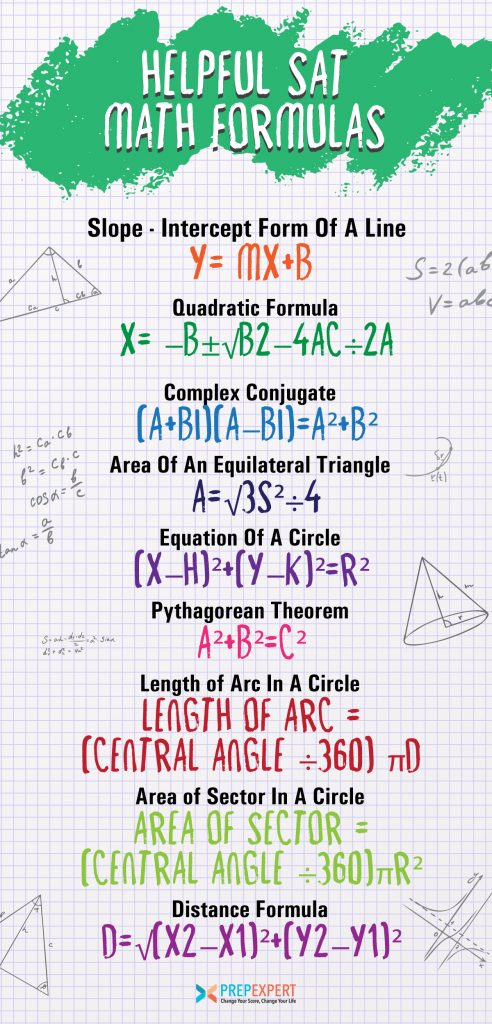One of the first things to do when studying for the SAT Math section is committing formulas to memory. If you’re not sure where to start, this list will help.

Here are 9 SAT math formulas that will help you out when taking the test. Use this list as a start.

Learn how to prepare for standardized tests with our year-round SAT prep and ACT prep courses today.

[sat_one]## Slope-Intercept Form Of A Line

### y=mx+b

m represents the slope of the equation,  “b represents the y-value of the y-intercept. For example, if we have the equation y=3x+5, then the slope is ‘3’ and the y-intercept is (0,5).

### x=−b±√b2−4ac÷2a

With this formula, you can find the roots of a quadratic equation if it isn’t easily factorable. However,  the quadratic has to be in the form of y=ax2+bx+c.

At that point, plug in the coefficients and constants into the formula. Remember, because of the ± in the numerator, you will have two separate answers.

## Complex Conjugate

### (a+bi)(a−bi)=a²+b²

Most SAT Math tests will have, at least, one question that requires handling imaginary numbers. The complex conjugate formula lets you cancel out the imaginary part of a complex number. As a result, you’ll be left with a real number.

## Area Of An Equilateral Triangle

### A=√3s²÷4

The formula for a regular triangle’s area is normally provided on the test’s reference sheet, but you need to know the triangle’s height. Sometimes you won’t be provided the height and have to figure it out.

The good news is you can calculate the area of an equilateral triangle by plugging the length of one of its sides into this formula. You can skip the height requirement!

## Equation Of A Circle

### (x−h)²+(y−k)²=r²

Normally, one SAT Math question will require using the equation of a circle. In this equation, the variables h and k represent the coordinate for the circle’s center and r

[sat_two]

## Pythagorean Theorem

### a²+b²=c²

The Pythagorean Theorem applies to regular right triangles. Its purpose is to let you solve for one of the unknown side lengths given any other side length. a and b are the triangle’s legs, and c is the hypotenuse.

## Length of Arc In A Circle

### length of arc = (central angle ÷360) πd

Geometry questions aren’t frequent in the SAT Math section, but you’ll probably still get a question asking about arcs or sectors in a circle. An arc is a length between two points on a circle.

They are usually measured by extending two radii from the circle’s center with an angle formed between them. You can use the degree measure of that arc as a fraction of 360 and then multiply it by the equation for the circumference to find the arc length.

## Area of Sector In A Circle

### area of sector = (central angle ÷360)πr²

Not unlike an arc, a circle’s sector is the area in between two radii extending from the center. Visually, think of it as a slice of pie basically. Again, multiply the degree measure as a fraction of 360 and then multiply it by the equation for a circle’s area to find the area of the sector.

## Distance Formula

### d=√(x2−x1)²+(y2−y1)²

The distance formula is fantastic for determining the distance between two points fast. To use it, simply take the coordinate values and plug them in to find the distance. and be sure to apply the squares and the square root at the right step.

[sat_three]

For more test strategy, college admissions, and scholarship application tips sign up for our FREE class happening right now!